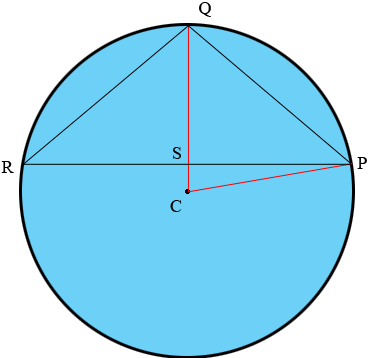SEARCH HOMEMath Central Quandaries & QueriesQuestion from NIHAL, a student: A isosceles triangle is inscribed in a circle having sides 20cm,20cm,30cm. find the radius of circleHi,

In my diagram $PQR$ is the isosceles triangle, C is the centre of the circle. Angle $QSP$ is a right angle. Can you see why?Use the fact that triangle $QSP$ to find the length $|QS|.$

Suppose that $r$ is the radius of the circle and $|SC| = x.$ Use the fact that $|QC| = r = |QS| + |SC|$ and the fact that triangle $PSC$ is a right triangle to find $r.$

PennyMath Central is supported by the University of Regina and the Imperial Oil Foundation.